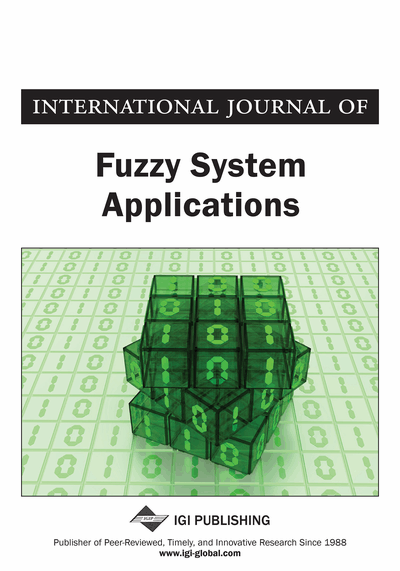# Chance Hypotheses Testing

S. Sampath (University of Madras, Chepauk, India) and L. Pephine Renitta (University of Madras, Chepauk, India)
DOI: 10.4018/IJFSA.2016070105

## Abstract

This paper considers the problem of testing hypotheses about hybrid distributions which are models representing situations where impreciseness (explained through fuzzy measure) and randomness (explained through probability measure) coexist. A criterion similar to the Neyman-Pearson criterion is proposed for testing a simple chance null hypothesis against a simple chance alternative hypothesis. The suggested criterion has been applied for testing hypotheses about hybrid triangular Bernoulli distribution and hybrid Poisson distribution. Optimal properties of the resulting tests have also been investigated.
Article Preview
Top

## 1. Introduction

Probability Theory is a highly popular subject which forms the basis of various branches of Statistics. This subject remains as a fascinating field of interest for hundreds of researchers. Contributions by researchers in different dimensions of the subject led to the development of tools meant for understanding and studying various aspects of uncertainty created by random phenomena. It is to be recalled that, as observed by Liu and Liu (2002) almost in all experimental studies, uncertainty enters into the picture not only through randomness but also in the form of impreciseness (also known as fuzziness). Existing theoretical ideas and available tools in probability theory are not sufficient to handle such situations. Sensing this inadequacy, Liu and Liu (2002) developed a new branch of study called Credibility Theory to explore and understand the functioning of systems under imprecise situations.

Researchers working in Credibility theory study various aspects which are analogues of those available in Probability theory. For example, several credibility distributions are being developed. Their properties and applications are also investigated by them. It is to be mentioned that the credibility distributions can be compared with probability distributions which are nothing but stochastic cum mathematical representations of random situations arising in real life scenario. Equipossible, Triangular, Trapezoidal, Normal and Exponential credibility distributions are some examples of credibility distributions which are mathematical representations explaining different forms of imprecise situations. For more details on such distributions one can refer to Liu (2008). Realizing the need for studies on credibility theory which are parallel to similar studies under Statistical Theory of Testing of Hypotheses, Sampath and Ramya (2015) have initiated studies on developing test procedures for testing hypotheses about credibility distributions. It is important to mention that Sampath and Ramya (2015) introduced a criterion called membership ratio criterion for testing credibility hypothesis and applied the same for testing credibility hypothesis about fuzzy triangular distribution. Using the suggested criterion, Sampath and Ramya (2014) made a study about credibility hypothesis testing of the expectation of fuzzy normal distribution. A study on the existence of optimal tests for testing credibility hypothesis about fuzzy exponential distribution has been done by Sampath and Ramya (2015). In this connection, it is to be recalled that prior to Liu’s Credibility theory, Zadeh (1965) introduced Fuzzy Set Theory for understanding imprecise situations. Studies on Testing of hypotheses about imprecise quantities based on the approach of Zadeh (1965) have been made. For example, Arnold (1996) presented an approach for testing fuzzily formulated hypotheses with crisp data, Filzmoser and Viertl (2004) presented an approach for statistical testing by using the characterizing function of the fuzzy-values and Torabi and Mirhosseini (2009) gave the Neyman-Pearson lemma to test fuzzy hypotheses. The concept of fuzzy test statistic usingcuts was given by Arefi and Taheri (2011). Testing fuzzy hypothesis with fuzzy data has been discussed by many researchers including Grzegorzewski (2002), Kruse and Meyer (1987) and Taheri and Behboodian (2006).

## Complete Article List

Search this Journal:
Reset
Open Access Articles: Forthcoming
Volume 9: 4 Issues (2020): 1 Released, 3 Forthcoming
Volume 8: 4 Issues (2019)
Volume 7: 4 Issues (2018)
Volume 6: 4 Issues (2017)
Volume 5: 4 Issues (2016)
Volume 4: 4 Issues (2015)
Volume 3: 4 Issues (2013)
Volume 2: 4 Issues (2012)
Volume 1: 4 Issues (2011)
View Complete Journal Contents Listing